# RANDOM TREES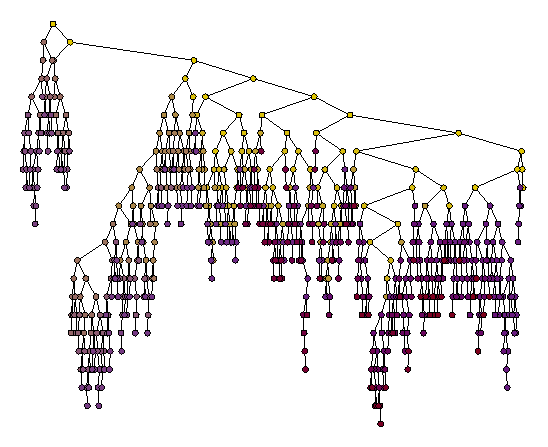I analyze various models of random trees. Even when the analysis seems to call for number theoretical or combinatorial tools, it turns out that good old probability theory often yields the shortest and most intuitive answers. The tree on the left is a random binary search tree for a normalized random walk sequence. Visit my random forest made for Bruce Reed for a poster at the CRM in Montreal.
 Take any nice mathematical sequence and construct a binary search tree. Then play with the angles and lengths of the edges a bit, and you'll obtain fascinating pictures in just a few lines of code. The figure on the right is based on (e), (2e), (3e), etcetera, where (.) denotes "modulo 1". For 3-d versions, see the 3-d Weyl trees made by Sylvain Bouix at McGill University.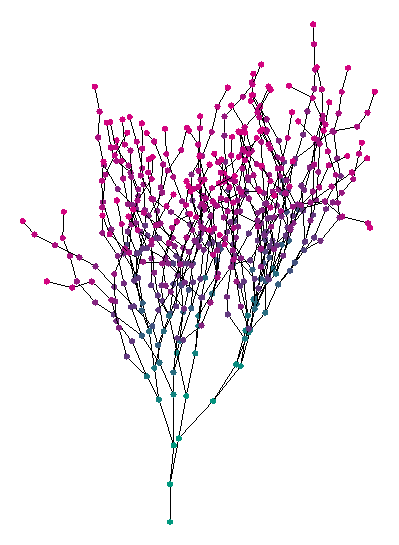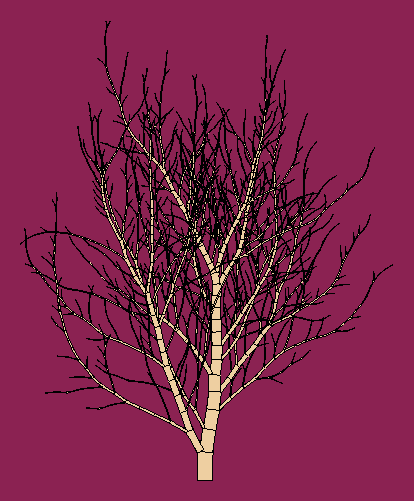Random trees based upon probabilistic models may be used in virtual scenes and offer a flexibility often not seen with fractal methods. The tree shown here is based upon random splitting of subtree sizes according to the beta distribution.
 A random Pythagoras tree. More trees here.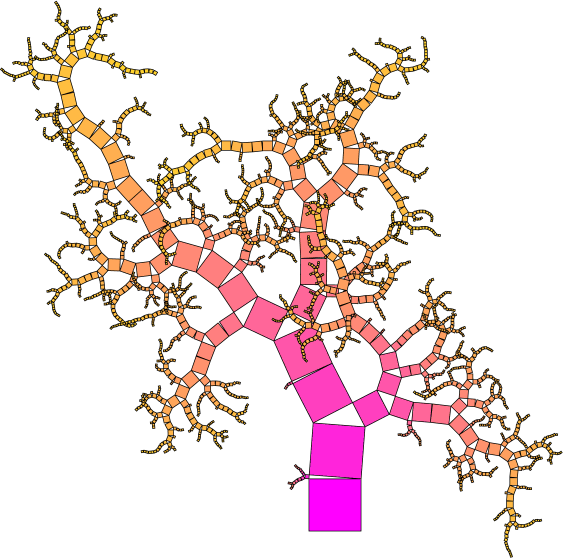A fern generated by a simple recursive PostScript program based upon fractals. All trees shown here are obtained by directly coded PostScript programs.A drawing of binary search trees in which the directions of child nodes alternate between horizontal and vertical, and the edge lengths decrease as 1 over square root of 2. The trees are Weyl trees (see paper below) for (na) mod 1, where a is from top to bottom, pi, e, square root of 19, (1/2) (1+ square root of 5), and at bottom, it is for n log n mod 1. The PostScript drawing was generated by P. van de Wal and Michel Dekking from the Technical University of Delft.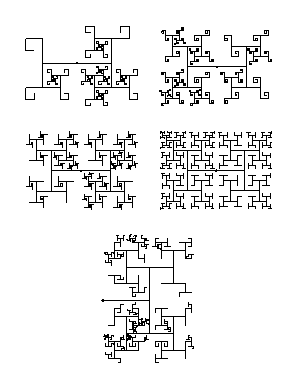Contact Luc Devroye School of Computer Science McGill University Montreal, Canada H3A 2K6 lucdevroye@gmail.com http://luc.devroye.org/index.html